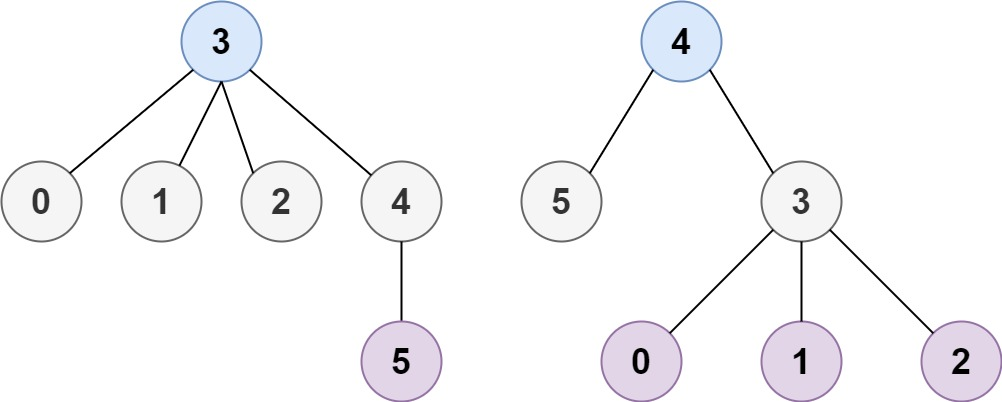# 最小高度树

## 310. 最小高度树 (Medium)```输入：n = 4, edges = [[1,0],[1,2],[1,3]]```输入：n = 6, edges = [[3,0],[3,1],[3,2],[3,4],[5,4]]

```

```输入：n = 1, edges = []

```

```输入：n = 2, edges = [[0,1]]

```

• `1 <= n <= 2 * 104`
• `edges.length == n - 1`
• `0 <= ai, bi < n`
• `ai != bi`
• 所有 `(ai, bi)` 互不相同
• 给定的输入 保证 是一棵树，并且 不会有重复的边

### 相关话题

[深度优先搜索] [广度优先搜索] [] [拓扑排序]

### 相似题目

1. 课程表 (Medium)
2. 课程表 II (Medium)